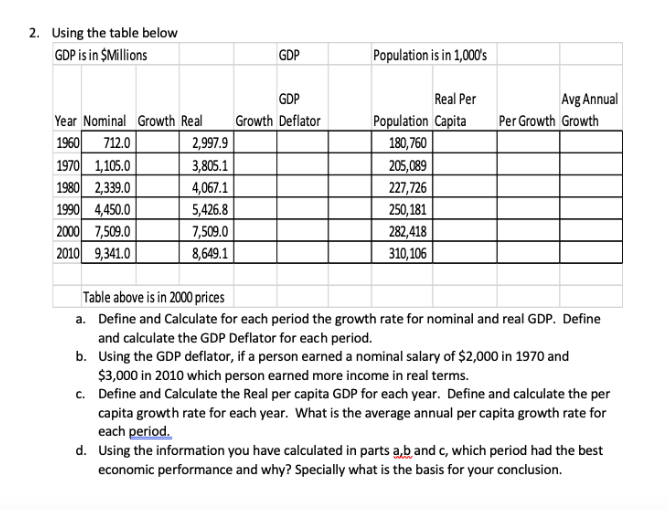# 2. Using the table belowGDP is in \$MillionsPopulation is in 1,000'sGDPAvg AnnualGDPReal PerPopulation CapitaGrowth DeflatorYear Nominal Growth RealPer Growth Growth1960 712.01970 1,105.01980 2,339.01990 4,450.02000 7,509.02010 9,341.02,997.9180,760205,0893,805.14,067.15,426.87,509.0227,726250,181282,418310,1068,649.1Table above is in 2000 pricesa. Define and Calculate for each period the growth rate for nominal and real GDP. Defineand calculate the GDP Deflator for each period.b. Using the GDP deflator, if a person earned a nominal salary of \$2,000 in 1970 and\$3,000 in 2010 which person earned more income in real terms.c. Define and Calculate the Real per capita GDP for each year. Define and calculate the percapita growth rate for each year. What is the average annual per capita growth rate foreach period.d. Using the information you have calculated in parts a,b and c, which period had the besteconomic performance and why? Specially what is the basis for your conclusion.

Question
163 views

Hello, I sent this assignment a couple days ago and it was rejected as being too complex, but I was wondering if at least one of each was demonstrated with the formula so I could do the others on my own.

Thank youhelp_outlineImage Transcriptionclose2. Using the table below GDP is in \$Millions Population is in 1,000's GDP Avg Annual GDP Real Per Population Capita Growth Deflator Year Nominal Growth Real Per Growth Growth 1960 712.0 1970 1,105.0 1980 2,339.0 1990 4,450.0 2000 7,509.0 2010 9,341.0 2,997.9 180,760 205,089 3,805.1 4,067.1 5,426.8 7,509.0 227,726 250,181 282,418 310,106 8,649.1 Table above is in 2000 prices a. Define and Calculate for each period the growth rate for nominal and real GDP. Define and calculate the GDP Deflator for each period. b. Using the GDP deflator, if a person earned a nominal salary of \$2,000 in 1970 and \$3,000 in 2010 which person earned more income in real terms. c. Define and Calculate the Real per capita GDP for each year. Define and calculate the per capita growth rate for each year. What is the average annual per capita growth rate for each period. d. Using the information you have calculated in parts a,b and c, which period had the best economic performance and why? Specially what is the basis for your conclusion. fullscreen
check_circle

Step 1

Hello. Since your question has multiple sub-parts, we will solve first three sub-parts for you. If you want remaining sub-parts to be solved, then please resubmit the whole question and specify those sub-parts you want us to solve.

a.

Gn is the growth rate of nominal GDP over a decade

Rn is the growth rate in real GDP over a decade

GD is the GDP Deflator

Nx is the nominal GDP in present year

Nx-1 is the nominal GDP in the previous year

Rx is the real GDP in present year

Rx-1 is the real GDP in previous year

Step 2

For 1960

GD = (712/2997.9) *100

23.75%

We cannot calculate Gn and Rn for year 1960 because we don’t have values for previous year.

For 1970

Gn = ((1105 - 712) / 712)*100

= 55.20%

Rn = ((3805.1 – 2997.9) / 2997.9) *100

= 26.93%

GD = (1105/3805.1)*100

=29.03%

The values for Gn, Rn, and GD can be calculated in similar manner for rest of the years.

Step 3

b.

For 1970 the GDP Deflator is 29.03% or 0.29. Then the salary in real terms is 0.29*2000 which is 580 that is less than his nominal salary.

GDP Deflator in 2010 is:

= (9341/8649.1)*100

...

### Want to see the full answer?

See Solution

#### Want to see this answer and more?

Solutions are written by subject experts who are available 24/7. Questions are typically answered within 1 hour.*

See Solution
*Response times may vary by subject and question.
Tagged in